| my account | login-logout | resources | support | catalog | home | get webcard |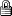Topic Closed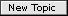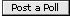Topic Closed search | help desk | commons
 » Online Classroom   » Inland and Coastal Navigation   » Public Discussion of Inland and Coastal Navigation   » How to do a running fix numerically (5-14)

Author Topic: How to do a running fix numerically (5-14)
 David Burch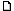posted April 05, 2004 11:52 AM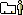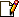A numerical running fix is best done by the method of distance off by two bow angles. We will use our practice problem no 5-14a as an example. Our course 085T, our speed 6 kts. A bearing to a buoy is 043T and then 20 minutes later the bearing is now 341T. Question, what is our distance off the buoy at the second sight? We have the bearing, so bearing plus distance is a fix.The first bow angle is 085-043 = 42° and the second bow angle is 085-341 = 85 (back to 000) and then 360-341 to the left of 000 = 19. Hence the second bow angle is 85+19= 104°. In other words, at the time of the first sight we would look dead ahead and then turn 42° to the left to see the buoy, then 20 minutes later we had to turn 104° to the left of the bow to see it.Now we need distance run between sights. At 6 kts, 20 minutes is 2 miles. So we have bow angle 42, run 2.0 miles and bow angle is 104. Question, how far away was that target?You can look this up in bowditch, or compute it. In bowditch it is table 18, from which you will find that the distance off is 0.76 times the distance run, or 0.76 x 2.0 = 1.52 nmi.To do this with the StarPilot, from the main menu choose Utilities, then choose Piloting, then select Distance off by two bearings, enter 042 and 104 and get 1.5 nmi.==========If you have a current set or leeway or both involved, you work the problem the same way, but the first step is to compute the corrected course and distance between sights. After that, all is the same.Example: problem 5-15c. Same as the above C=085, S=6.0, bearing one = 042, bearing two = 341, twenty minutes later, but now we are in a current of 1.0 kts to the south and with a north wind giving us a 7° leeway to the south.The leeway simply changes the 085 to 092. it is real motion through the water. so what is our CMG and our SMG sailing at 6 kts on heading 092 in 1 kt to the south. You can solve this with a vector triangle or use the StarPilot under current sailings: just enter C=092, S=6.0, set=180, drift=1.0, to get CMG=101.4 and SMG=6.12.Now we have bow angle one = 101.4 - 043 = 58.4° and bow angle two = 101.4 - 341 = 101.4 + 19 = 120.4­°.And we will need the distance run: ie 20 minutes at 6.12 = what? This we get from the StarPilot in one step = 2.04 nmiFrom Table 18, rounding to 58 and 120 we get dist off = 0.96 x 2.04 = 1.96 nm. With the starpilot we get 2.0 since for now we show only one decimal place in the answer.So it looks like our answer in the chart problems book for 5-15c of 2.25 nmi is not right. Should be just under 2.0.There is a related discussion of this elsewehere in this group.If there are questions about this process, please post them here. It is an excellent way to test your plotting.Note you can get a distance off computer in our NIMA calculator package, which is a free download in the Freeware section of our online Ressources Library. Or download it here:computers for distance by two bearings From: Starpath, Seattle, WA

 All times are Pacific